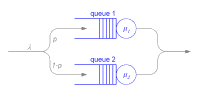sources:
generator.cpp (709 bytes)
generator.h (417 bytes)
generator_n.cpp (4.0k)
lbs_i.cpp (2.6k)
lbs_i.h (430 bytes)
lbs_i_n.cpp (4.1k)
lbs_ii.cpp (3.3k)
lbs_ii.h (439 bytes)
lbs_ii_n.cpp (4.1k)
minimumselector.cpp (1.7k)
minimumselector.h (359 bytes)
minimumselector_n.cpp (4.1k)
randomselector.cpp (454 bytes)
randomselector.h (266 bytes)
randomselector_n.cpp (4.1k)
server.cpp (1.6k)
server.h (508 bytes)
server_n.cpp (4.0k)
servicenet_n.cpp (13.6k)
sink.cpp (1.3k)
sink.h (495 bytes)
sink_n.cpp (3.9k)

website:```   1 #include "sink.h"   2   3 // Module registration:   4 Define_Module( Sink );   5   6 void Sink::activity()   7 {   8     // reset statistics   9     sInfo.clearResult();  10     totalCount = 0;  11  12     // get number of samples to record  13     int sampleCount = par("sample_count");  14  15     // file to write history to  16     cOutVector srt("system response time", 1);  17  18     // message loop  19     for(int i = 0; i<sampleCount; i++)  20     {  21         // receive a message and record time statistics  22         cMessage *msg = receive();  23         // create statistics  24         simtime_t msrt = simTime() - msg->timestamp();  25         sInfo.collect(msrt);  26         srt.record(msrt);  27         // delete msg  28         delete msg;  29     }     30     endSimulation();  31 }  32  33 void Sink::finish()  34 {  35     ev << "Total jobs processed: " << totalCount + sInfo.samples() << endl;  36     ev << "Number of jobs used for statistics: " << sInfo.samples() << endl;  37     ev << "Avg system response time: " << sInfo.mean() << endl;  38     ev << "Standard deviation: " << sInfo.stddev() << endl;  39     ev << "Max system response time: " << sInfo.max() << endl;  40     ev << "Min system response time: " << sInfo.min() << endl;  41  42     ev << endl;  43 }  44  45 void Sink::reset()  46 {  47     // update totalCount  48     totalCount += sInfo.samples();  49     sInfo.clearResult();  50 }  51 ```